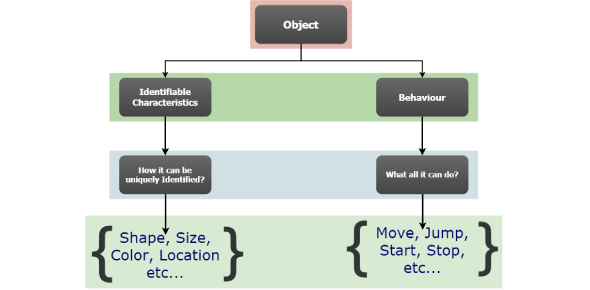# Python Classes And Objects Quiz

10 Questions | Attempts: 8940
ShareSettingsWant to test your coding prowess? Take this Python classes and objects quiz in order to see how good you actually are at Python and coding. Who knows, you might even learn something new! Python is a high-level, general-purpose programming language made to aid in the creation of both large and small-scale projects. It also emphasizes code readability through the use of an extensive indentation format. Share the quiz with your friends once you are done taking it so they can test themselves as well! All the best!

• 1.
For the following code, which of the following statements is true?   def printHello(): print("Hello") a = printHello()
• A.

PrintHello() is a function and a is a variable. None of them are objects.

• B.

Both printHello() and a refer to the same object.

• C.

PrintHello() and a refer to different objects.

• D.

Syntax error! You cannot assign function to a variable in Python.

• 2.
What is the output of the following program?   def outerFunction(): global a a = 20 def innerFunction(): global a a = 30 print('a =', a) a = 10 outerFunction() print('a =', a)
• A.

A = 10  a = 30

• B.

A = 10

• C.

A = 20

• D.

A = 30

• 3.
Which of the following statements is true?
• A.

A class is blueprint for the object.

• B.

You can only make a single object from the given class.

• C.

Both statements are true.

• D.

Neither statement is true.

• 4.
What is the output of the following code?   class Foo: def printLine(self, line='Python'): print(line) o1 = Foo() o1.printLine('Java')
• A.

Python

• B.

Line

• C.

Java

• D.

Java Python

• 5.
What does the __init__() the function do in Python?
• A.

Initializes the class for use.

• B.

This function is called when a new object is instantiated.

• C.

Initializes all the data attributes to zero when called.

• D.

None of the above.

• 6.
What is the output of the following code?   class Point: def __init__(self, x = 0, y = 0): self.x = x+1 self.y = y+1 p1 = Point() print(p1.x, p1.y)
• A.

0 0

• B.

1 1

• C.

None None

• D.

X y

• 7.
Which of the following code uses the inheritance feature of Python?
• A.

class Foo: Pass

• B.

class Foo(object): pass class Hoo(object): pass

• C.

class Foo: pass class Hoo(Foo): pass

• D.

None of the above code.

• 8.
If you a class is derived from two different classes, it’s called ______
• A.

Multilevel Inheritance

• B.

Multiple Inheritance

• C.

Hierarchical Inheritance

• D.

Python Inheritance

• 9.
Which of the following statements is true?
• A.

In Python, same operator may behave differently depending upon operands.

• B.

You can change the way operators behave in Python.

• C.

Special method __add()__ is called when + operator is used.

• D.

All of the above.

• 10.
What is the output of the following code?   class Point: def __init__(self, x = 0, y = 0): self.x = x self.y = y def __sub__(self, other): x = self.x + other.x y = self.y + other.y return Point(x,y) p1 = Point(3, 4) p2 = Point(1, 2) result = p1-p2 print(result.x, result.y)
• A.

2 2

• B.

4 6

• C.

0 0

• D.

1 1

## Related TopicsBack to top
×

Wait!
Here's an interesting quiz for you.